ofthisandthatQuick Quiz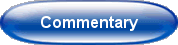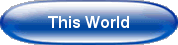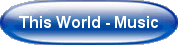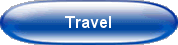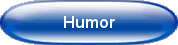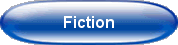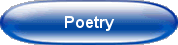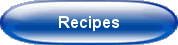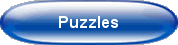@import url(http://www.google.com/cse/api/branding.css);Custom SearchMarch 24, 2014

PUZZLE -- GUESSING AGE PARTY GAME

than 10 years old).  Then you are asked to pick a number between 1 and 9, multiply
it by 9 and then subtract this product from your age multiplied by 10.  You tell the
'mind-reader' this difference, and he promptly guesses your age correctly.  He even
tells you the number (between 1 and 9) randomly chosen.  How?

(Scroll down for the solution)

Solution:  Whenever a two-digit number is multiplied by 10 and a single-digit number
multiplied by 9 is subtracted, the first digit of the difference has to be the single-digit
number.  Thus

Difference = 10 Age - 9 Number
= 10 Age - 10 Number + Number

10 Age - 10 Number = Difference - Number

Age = (Difference - Number)/10 + Number

Example:

Age = 25, Number = 3

10(25) - 9(3) = 223

Thus the number chosen is 3 and the age is 22 + 3 = 25

Confirmation from formula:

Age = (Difference - Number)/10 + Number

= [(223 - 3) /10] + 3 = 22 + 3 = 25